Bonky Zhu
If someone is able to show me that what I think or do is not right, I will happily change, for I seek the truth, by which no one was ever truly harmed. It is the person who continues in his self-deception and ignorance who is harmed.

AliMe Chat：基于Seq2Seq和重排的聊天机器人

1. 序言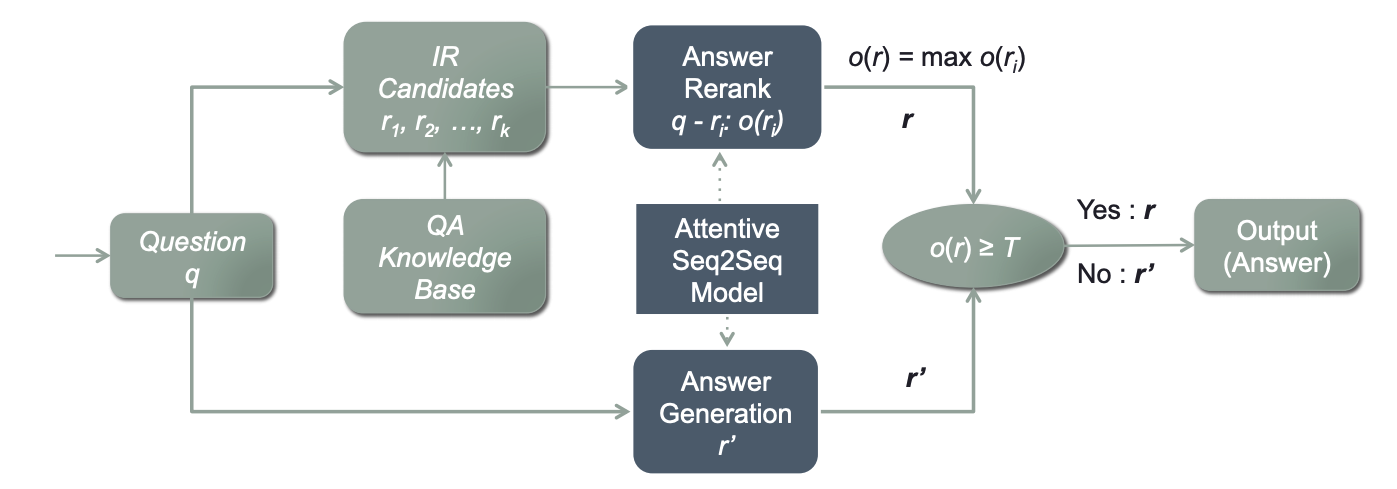• 我们提出了一种新颖的混合方法，利用一个序列到序列模型来优化IR和生成模型的联合结果。
• 我们进行了一系列的实验来评估这种方法。结果表明，我们方法在IR和生成两方面表现都很出色。
• 我们将我们的聊天机器人引擎和一个公共的聊天机器人进行了比较。证据表明我们的引擎性能更好。
• 我们启用了AliMe聊天，用于真实的工业应用。

2. 基于Seq2Seq的重排模型

1. 这三种模式都是基于单词的（即需要分词）：IR模型的输入特征是单词，而生成模型和重排模型的输入特征是单词向量表示，这些都是使用fasttext预先训练和在两个模型中进行进一步调整的
2. 我们的生成模型和重排模型是建立于同一序列到序列结构，前者生成输出，后者则是岁输入问题的候选答案进行评分。

1. 首先，我们使用IR模型检索一组$k$个候选的QA对。

$$\left\langle q_{\mathrm{kb}{i}}, r{i}\right\rangle_{i=1}^{k}(k=10)$$

1. 我们将每个问题$q$与其候选答案$r_i$配对 ，并为每一个问答对使用重排模型中的计分公式计算置信度得分

$$o\left(r_{i}\right)=s\left(q, r_{i}\right)$$

1. 我们考虑答案$r$使用最大分数$o(r)=\max o\left(r_{i}\right)$:如果$o(r) ≥T$，采取答案$r$；否则输出一个基于生成模型的回复$r’$。

2.3 生成模型

\begin{aligned} p\left(y_{i}=w_{i} | \theta_{i}\right) &=p\left(y_{i}=w_{i} | y_{1}, y_{2}, \ldots, y_{i-1}, c_{i}\right) \ &=f\left(y_{i-1}, s_{i-1}, c_{i}\right) \end{aligned}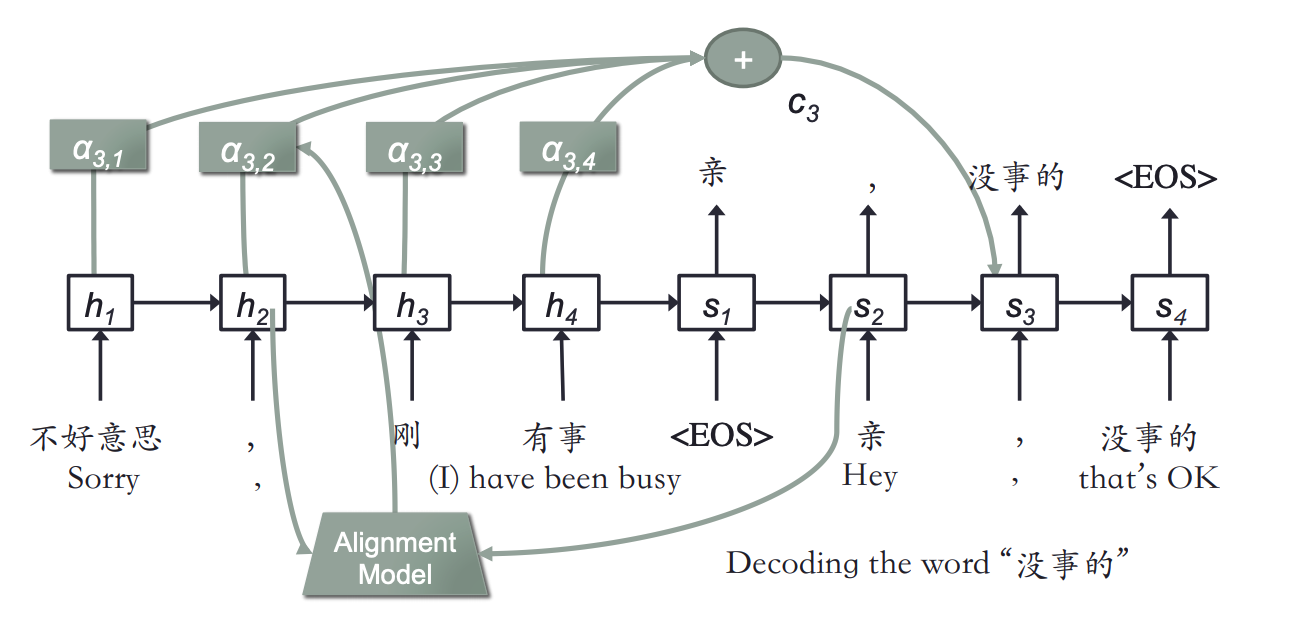Bucketing and padding. 为了处理不同长度的问题和答案，我们采用了在Tensorflow中提出的存储桶机制。我们使用五个存储桶 (5, 5), (5, 10), (10, 15), (20, 30),(45, 60)来容纳不同长度的QA对，例如，长度为4的问题和一个长度为8的答案将被放在存储桶(5, 10)，并在需要时使用特殊符号“PAD”填充问题和答案。

Softmax over sampled words. 为了加快训练过程，我们将softmax应用于一组抽样词汇（目标单词加上512随机单词），而不是整个集合。这个想法与的重要性抽样策略相似。

Beam search decoder. 在解码相位，我们使用定向搜索，在每个时刻 t 维持 top-k (k = 10) 输出序列，而不是贪婪搜索，每个时刻 t 只保留一个，以使我们的生成更加合理。

2.4 序列到序列重排模型

$$s^{\text {Mean-Prob }}=\frac{1}{n} \sum_{i=1}^{n} p\left(y_{i}=w_{i} | \theta_{i}\right)$$

3 实验

3.1 评估重排模型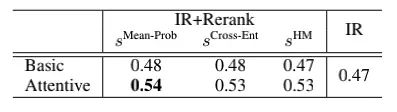3.2 评估候选方法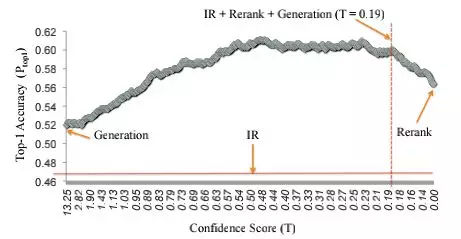3.3 线上 A/B测试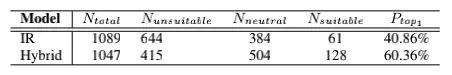Share